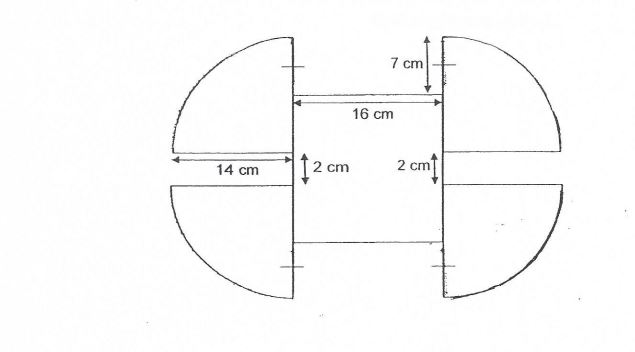# Question 1 of 84

The figure below is made up of 4 identical quadrants and a square. Find The area of the figure. $(Take \pi = \frac{22}{7})$A
868cm$^2$
B
870cm$^2$
C
872cm$^2$
D
874cm$^2$
E
None of the above